Function Repository Resource:

# GramianReduce

Find a unimodular conversion matrix corresponding to a lattice Gramian matrix

Contributed by: Daniel Lichtblau
 ResourceFunction["GramianReduce"][mat] treats mat as the Gram matrix of an integer lattice and returns matrices {u,b} where u is unimodular (invertible over the integers) and b satisfies b==u.mat.Transpose[u].

## Details and Options

A Gram matrix for a lattice given by generating vectors V={v1,v2,} is the matrix of inner products V.Vt.
The matrix mat should contain only integers or rationals.
Gram matrices are symmetric and positive definite.
ResourceFunction["GramianReduce"] treats its input as symmetric and positive definite without regard to how it might be decomposed into a product of a lattice and its transpose.
Given an actual lattice, one can similarly obtain a unimodular transformation matrix for its Gramian using the resource function ExtendedLatticeReduce.

## Examples

### Basic Examples (3)

Reduce a 3×3 Gramian matrix:

 In:=Out=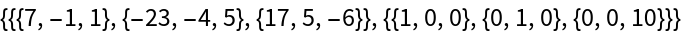Check unimodularity:

 In:=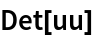Out=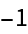Check the matrix identity:

 In:=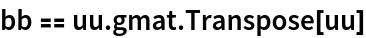Out=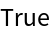### Properties and Relations (6)

Reduce a larger Gramian:

 In:=In:=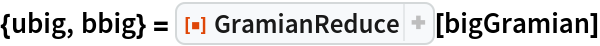Out=Check that the transformation matrix unimodularity and the matrix product identity properties both hold:

 In:=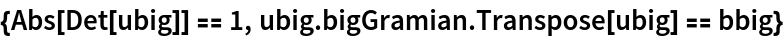Out=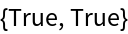This matrix is the Gramian of a certain matrix:

 In:=Out=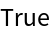Compute the lattice reduction and unimodular transformation of this lattice using the resource function ExtendedLatticeReduce:

 In:=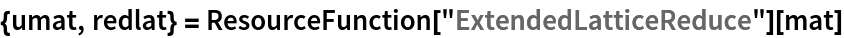Out=The transformation is the same as the one obtained by GramianReduce:

 In:=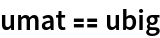Out=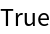In this example, the reduced lattice also gives rise to the reduced Gramian:

 In:=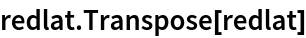Out=## Version History

• 1.0.0 – 17 January 2020

## Author Notes

A different Wolfram Language implementation by Wilberd van der Kallen can be found at the links below: